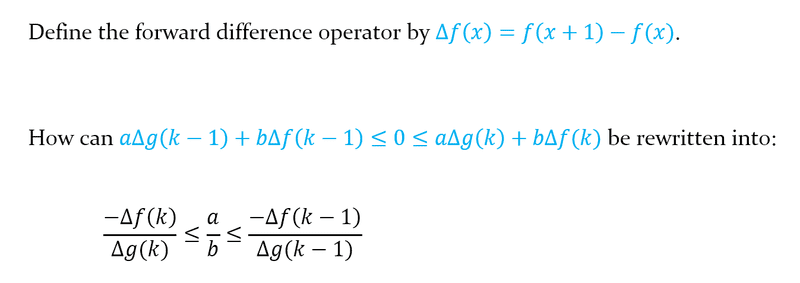# Help with rewriting a compound inequality

• I
Andrea94
TL;DR Summary
Help with rewriting optimality conditions for integer-convex functions.
See attached screenshot.
Stumped on this, I'll take anything at this point (hints, solution, etc).•PhDeezNutz

Homework Helper
Big problems are to be split into smaller problems. You have two inequalities. Write the first one down and manipulate ! Then idem number two.

##\ ##

•Andrea94
Homework Helper
And: what does integer-convex mean for e.g. $$g(k+1) + g(k-1) - 2g(k) \quad \textsf{?}$$

##\ ##

Andrea94
Big problems are to be split into smaller problems. You have two inequalities. Write the first one down and manipulate ! Then idem number two.

##\ ##
Great, thanks! Didn't even think about the fact that I could do each inequality separately.

Andrea94
And: what does integer-convex mean for e.g. $$g(k+1) + g(k-1) - 2g(k) \quad \textsf{?}$$

##\ ##
What do you mean? The expression you've written is the same thing as $$\Delta g(k) - \Delta g(k-1)$$ but I'm not sure how that is relevant.

Homework Helper
What do you mean? The expression you've written is the same thing as $$\Delta g(k) - \Delta g(k-1)$$ but I'm not sure how that is relevant.
Right, but ##\Delta g(k) - \Delta g(k-1)## can be ##\ge 0## or ##\le 0## for an integer-convex function ... whereas ...##\ ##

Andrea94
Right, but ##\Delta g(k) - \Delta g(k-1)## can be ##\ge 0## or ##\le 0## for an integer-convex function ... whereas ...##\ ##
Still not sure what I'm supposed to see here 😅

Homework Helper
The problem with inequalities is that you can only multiply (or divide) left and right with something positive. As it happens, for integer-convex functions the sign of ##g(k+1) + g(k-1) - 2g(k)## is ...

##\ ##

Andrea94
The problem with inequalities is that you can only multiply (or divide) left and right with something positive. As it happens, for integer-convex functions the sign of ##g(k+1) + g(k-1) - 2g(k)## is ...

##\ ##
Hm, the only thing I can think of is that if ##k## is optimal and nonzero, then ##g(k+1) + g(k-1) - 2g(k)## is always positive since for optimal ##k## we have ##\Delta g(k) > 0## and ##\Delta g(k-1) < 0##. Is this what you mean?

Homework Helper
Not sure where your 'optimal' comes from (it seems to live in your context, but not in the context of this thread ?).

But: yes, for a convex function the second derivative is always positive and so is this ##g(k+1) + g(k-1) - 2g(k)##.

I figured it might help in manipulating the inequalities ...

##\ ##

•Andrea94
Andrea94
Not sure where your 'optimal' comes from (it seems to live in your context, but not in the context of this thread ?).

But: yes, for a convex function the second derivative is always positive and so is this ##g(k+1) + g(k-1) - 2g(k)##.

I figured it might help in manipulating the inequalities ...

##\ ##
Ohh I see, thanks a lot for the help!